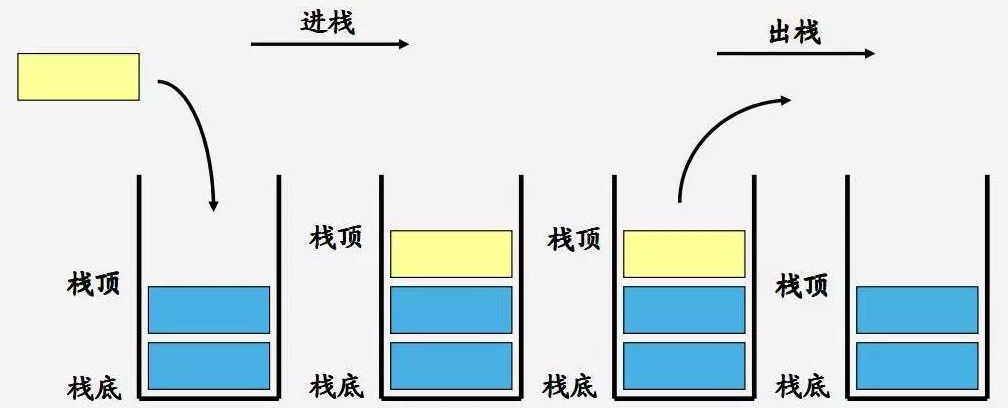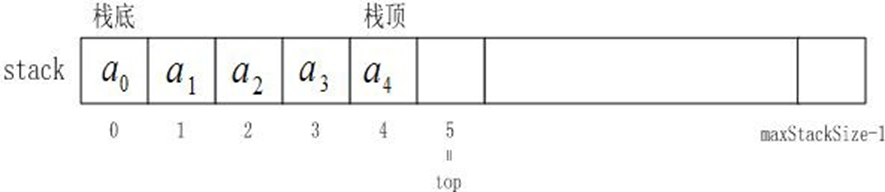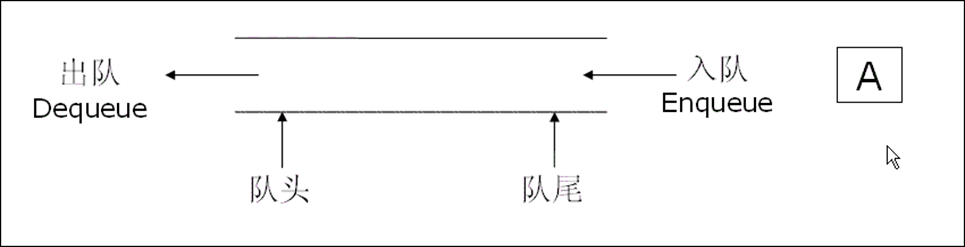# 1.栈

## 1.1栈的概念及结构## 1.2栈的实现``````typedef int STDataType;
#define N 10
typedef struct Stack
{
STDataType _a[N];
int _top; // 栈顶
}Stack;
``````

``````// 支持动态增长的栈
typedef int STDataType;
typedef struct Stack
{
STDataType* _a;
int _top; // 栈顶
int _capacity; // 容量
}Stack;
// 初始化栈
void StackInit(Stack* ps);
// 入栈
void StackPush(Stack* ps, STDataType data);
// 出栈
void StackPop(Stack* ps);
// 获取栈顶元素
STDataType StackTop(Stack* ps);
// 获取栈中有效元素个数
int StackSize(Stack* ps);
// 检测栈是否为空，如果为空返回非零结果，如果不为空返回0
int StackEmpty(Stack* ps);
// 销毁栈
void StackDestroy(Stack* ps);
``````

# 2.队列

## 2.1队列的概念及结构## 2.2队列的实现

``````// 链式结构：表示队列
typedef struct QListNode
{
struct QListNode* _pNext;
QDataType _data;
}QNode;
// 队列的结构
typedef struct Queue
{
QNode* _front;
QNode* _rear;
}Queue;
// 初始化队列
void QueueInit(Queue* q);
// 队尾入队列
void QueuePush(Queue* q, QDataType data);
// 队头出队列
void QueuePop(Queue* q);
// 获取队列头部元素
QDataType QueueFront(Queue* q);
// 获取队列队尾元素
QDataType QueueBack(Queue* q);
// 获取队列中有效元素个数
int QueueSize(Queue* q);
// 检测队列是否为空，如果为空返回非零结果，如果非空返回0
int QueueEmpty(Queue* q);
// 销毁队列
void QueueDestroy(Queue* q);
``````

# 3.栈和队列面试题

（C语言）数据结构之栈和队列面试题